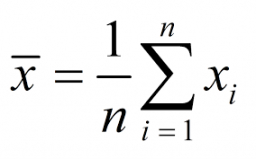# Weighted-average

Calculate the weighted-average cost of the following inventory purchases:

Date Quantity Purchased Cost per Unit
May 4 33 $12.25 May 11 41$13.87
May 29 37 \$11.99

a =  12.7617 USD

### Step-by-step explanation:Did you find an error or inaccuracy? Feel free to write us. Thank you!

Tips for related online calculators
Looking for help with calculating arithmetic mean?
Looking for a statistical calculator?
Need help calculating sum, simplifying, or multiplying fractions? Try our fraction calculator.

#### Grade of the word problem:

We encourage you to watch this tutorial video on this math problem: